# Biochemistry : Other Kinetics Principles

## Example Questions

### Example Question #1 : Other Kinetics Principles

Suppose that an enzyme has a critical functional group in its active site that is heavily involved in carrying out the catalytic mechanism. To perform its role in catalyzing its target reaction, this particular functional group must be able to accept a proton from the intermediate during the process. If the pKa of this functional group is equal to 7.0, then what percentage of the total enzyme active sites for this enzyme would be in the active form in a solution in which the pH is equal to 6.4?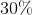Explanation:

In order to solve this problem, we'll need to make use of the Henderson-Hasselbalch equation.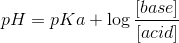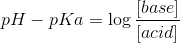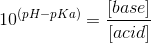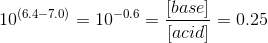Therefore, for every 0.25mol of base (deprotonated functional group), there is 1mol of acid (protonated functional group). Furthermore, we're told in the question stem that the functional group must be able to accept a proton from the intermediate during the catalytic mechanism. To accept a proton, the functional group would need to be in its deprotonated form to be active. Hence, we're trying to find the percentage of the deprotonated form. To find this value, we'll use the following expression: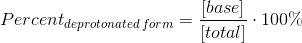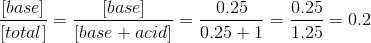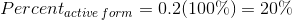### Example Question #41 : Enzyme Kinetics And Models

Which of the following does not increase reaction rate?

Increasing temperature

Increasing the concentration of substrates

Introducing cofactors

Decrease amount of catalysts

Optimizing pH

Decrease amount of catalysts

Explanation:

Catalysts lower activation energy. Lowering activation energy causes the reaction rate to increase. Removing catalysts will cause the reaction to slow down because the activation energy will be higher. Cofactors assist in the function of enzymes and can increase reaction rate.

### Example Question #42 : Enzyme Kinetics And Models

There are at least four types of glucose transporter in the body. GLUT1 and GLUT3 are located in most tissues including the brain and the red blood cells. These glucose transporters rapidly take up glucose from the blood but have the lowest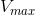value. GLUT2 is commonly found in the liver and the pancreas. GLUT2 has a lower affinity for glucose but has the highestvalue. GLUT4 is common in skeletal tissues and in adipose tissues. This transporter is normally not active for uptake unless stimulated by insulin or during exercise.

What is the mechanism by which the GLUT proteins in the transport glucose into the cell from the blood?

Active transport

Facilitated diffusion

Antiport

Symport

Voltage-gated channel

Facilitated diffusion

Explanation:

Since there will be more glucose surrounding the cell, the GLUT proteins utilize a chemical gradient to transport glucose into the cell.

### Example Question #721 : Biochemistry

If the level of ATP suddenly increases in a cell, facilitated diffusion in the cell will __________.

remain unchanged

decrease instantly

decrease slowly

increase slowly

increase instantly

remain unchanged

Explanation:

Facilitated diffusion acts independently of the level of intracellular ATP. Therefore, a change in ATP concentration will not affect the rate of facilitated diffusion.

### Example Question #51 : Enzyme Kinetics And Models

You make a Hill plot reflecting the binding kinetics of a receptor and see that the slope (the Hill coefficient) is 1. What does this indicate about your receptor?

Binding is positively cooperative

Binding is negatively cooperative

Binding is non-cooperative

The reaction is proceeding at a rate ofThe reaction is proceeding at a rate of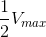Binding is non-cooperative

Explanation:

If the Hill coefficient of the Hill plot is equal to 1, then binding is non-cooperative. If the Hill coefficient is greater than 1, binding is positively cooperative; if less than 1, binding is negatively cooperative. The Hill plot does not make any conclusions about the rate of a reaction, which involves Michaelis-Menten kinetics.

### Example Question #52 : Enzyme Kinetics And Models

Given the following kinetic parameters, which of the following enzymes would show the most efficiency?

An enzyme with a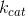value of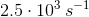and a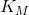value of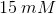An enzyme with avalue of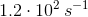and avalue of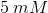An enzyme with avalue of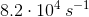and avalue of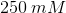An enzyme with avalue of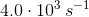and avalue of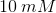An enzyme with avalue of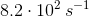and avalue of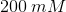An enzyme with avalue ofand avalue ofExplanation:

To answer this question, we need to understand what enzyme efficiency is and how it's calculated.

Enzyme efficiency refers to how much substrate a given enzyme can convert into product for a given amount of substrate. In other words, an enzyme that is very efficient can convert substrate into product very quickly, even when there is not very much of that substrate around.

To calculate an enzyme's efficiency, we need to take into account these two factors by considering the variables they are associated with.

Thefor a given enzyme represents the "turnover number." This value is a rate constant that is unique to a particular enzyme at a certain temperature (generally under physiological conditions). This rate constant refers to the maximum amount of substrate that an enzyme can convert into product in a given amount of time. Or, put another way, the rate constant gives the maximum reaction rate for a particular enzyme when that enzyme is completely saturated with substrate.

On the other hand, theof an enzyme tells us the amount of substrate that needs to be present in order for the reaction rate to be at exactly half of its maximal value. This is almost the same as saying how much attraction a given enzyme has for its substrate. In fact, under certain conditions, we can use theof an enzyme as an accurate measure of the affinity that the enzyme has for its substrate.

Relating these two values back to enzyme efficiency, we can calculate its value by taking the ratio of the two. In other words,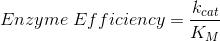So, the greater theand the lower the, the greater the enzyme's efficiency will be.

From the answer choices shown, we can see that the ratio is greatest for the enzyme that has aofand aof, which gives an efficiency value of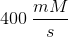.

### Example Question #2 : Other Kinetics Principles

If the chloride concentration is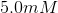in the cell and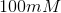in the blood, what is the electrochemical potential of chloride ions across the plasma membrane at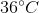when the electrical potential across the membrane is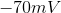and the inside is negative?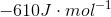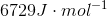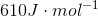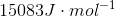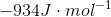Explanation: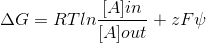Whereis Faraday's constant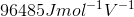,is the charge of a chloride ion, which is, and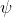isor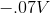. Be sure to keep units consistent.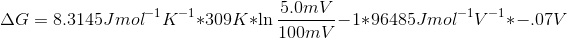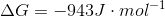### Example Question #54 : Enzyme Kinetics And Models

Which of the following coenzymes functions primarily by transferring one-carbon groups?

Pyridoxal phosphate

Thiamine pyrophosphate

Explanation:

This question is asking us to identify a coenzyme that functions by transferring one-carbon groups. So let's take a look at each answer choice to determine what it does.

Nicotinamide Adenine Dinucleotide (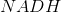) and Flavin Adenine Dinucleotide (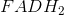) both function as carriers of high-energy electrons. Both of these coenzymes are heavily involved in taking high-energy electrons from various compounds as they are broken down during catabolic reactions. Once collected, these coenzymes deposit their high-energy electrons into the electron transport chain, allowing for a great deal of ATP to be generated for energy.

Pyridoxal Phosphate (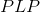) is a coenzyme that is primarily involved in transamination reactions. These are reactions that take an amino group from amino acids, and transfer that amino group to an alpha-keto acid, converting it into an amino acid in the process. Thus, this coenzyme transfers amino groups.

Thiamine Pyrophosphate (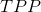) is a coenzyme responsible for transferring two-carbon groups. For instance,serves as a coenzyme for the pyruvate dehydrogenase complex, which is responsible for converting pyruvate into acetyl-CoA.

Finally, S-Adenosyl Methionine (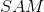) is a coenzyme mainly responsible for transferring one-carbon groups in their most reduced form, as methyl groups. As the most potent methyl group donor in biological systems,functions as a coenzyme for many methyltransferase enzymes.

### All Biochemistry Resources